Courses

# Ex 12.1 NCERT Solutions- Heron’s Formula Class 9 Notes | EduRev

## Mathematics (Maths) Class 9

Created by: Full Circle

## Class 9 : Ex 12.1 NCERT Solutions- Heron’s Formula Class 9 Notes | EduRev

The document Ex 12.1 NCERT Solutions- Heron’s Formula Class 9 Notes | EduRev is a part of the Class 9 Course Mathematics (Maths) Class 9.
All you need of Class 9 at this link: Class 9

Question 1. A traffic signal board, indicating ‘SCHOOL AHEAD’, is an equilateral triangle with side ‘a’. Find the area of the signal board, using Heron’s formula. If its perimeter is 180 cm, what will be the area of the signal board?
Solution:
For an equilateral triangle with side ‘a’, area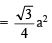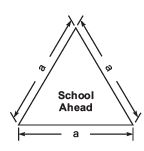∵ Each side of the triangle = a cm
∴ a + a + a = 180 cm
⇒ 3a = 180 cm
⇒ a= (180/3) = 60 cm
Now, s = Semi-perimeter = (180/2) = 90 cm

∴  Area of triangle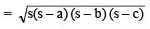∵  Area of the given triangle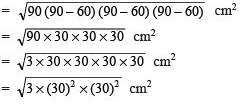= 30 x 30 x√3 cm
= 900 √3 cm
Thus, the area of the given triangle = 900√3cm2

Question 2. The triangular side walls of a flyover have been used for advertisements. The sides of the walls are 122 m, 22 m and 120 m (see Fig. ). The advertisements yield an earning of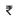5000 per m2 per year. A company hired one of its walls for 3 months. How much rent did it pay?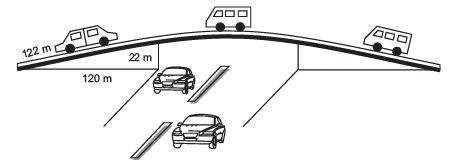Solution: ∵ The sides of the triangular wall are
a = 122 m, b = 120 m, c = 22 m
∴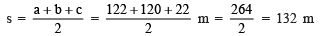∵ The area of a triangle is given by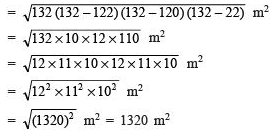∵ Rent for 1 year (i.e. 12 months) per m= ₹ 5000
∴ Rent for 3 months per m2 = ₹5000 x (3/12)
⇒ Rent for 3 months for 1320 m= ₹5000 x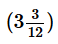x 1320
= ` 5000 x 3 x 110 = ₹16,50,000.

Question 3. There is a slide in a park. One of its side walls has been painted in some colour with a message “ KEEP THE PARK GREEN AND CLEAN” (See figure). If the sides of the wall are 15 m, 11 m and 6 m, find the area painted in colour.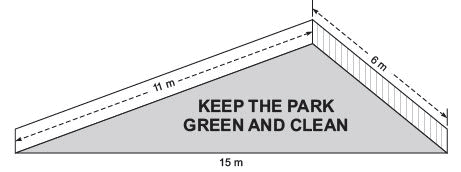Solution: ∵ Sides of the wall are 15 m, 11 m and 6 m.
∴   a = 15 m, b = 11 m, c = 6 m
∴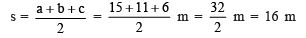Now, area of the triangular surface of the wall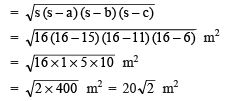Thus, the required area painted in colour = 20√2 m2

Question 4. Find the area of a triangle two sides of which are 18 cm and 10 cm and the perimeter is 42 cm.
Solution:
Let the sides of the triangle be a = 18 cm, b = 10 cm and c = ?
∵ Perimeter (2s) = 42 cm
⇒ s= (42/2)  = 21 cm
∴ c = 42 – (18 + 10) cm = 14 cm
∵ Area of a triangle =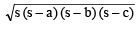∴ Area of the given triangle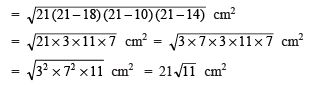Thus, the required area of the triangle = 21√11 cm

Question 5. Side of a triangle are in the ratio of 12 : 17 : 25 and its perimeter is 540 cm. Find its area.
Solution:
Perimeter of the triangle = 540 cm
⇒ Semi-perimeter of the triangle, s= (540/2)
= 270 cm
∵ The sides are in the ratio of 12 : 17 : 25.
∴ a = 12x cm, b = 17x cm, c = 25x cm
∴ 12x + 17x + 25x = 540
⇒ 54x = 540
⇒ x = (540/54) = 10
∴ a = 12 x 10 = 120 cm b = 17 x 10
= 170 cm c = 25 x 10
= 250 cm
⇒ (s – a) = (270 – 120) cm
= 150 cm (s – b)
= (270 – 170) cm
= 100 cm (s – c)
= (270 – 250) cm
= 20 cm
∴ Area of the triangle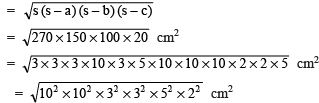= 10 x 10 x 3 x 3 x 5 x 2 cm2 = 9,000 cm2
Thus, the required area of the triangle = 9,000 cm2

Question 6. An isosceles triangle has perimeter 30 cm and each of the equal side is 12 cm. Find the area of the triangle.
Solution
: Equal sides of the triangle are 12 cm each.
Let the third side = x cm.
Since, perimeter = 30 cm
∴ 12 cm + 12 cm + x cm = 30 cm
⇒ x = 30 – 12 – 12 cm
⇒ x = 6 cm
Now, semi-perimeter = (30/2)cm = 15 cm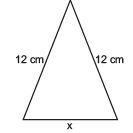∴ Area of the triangle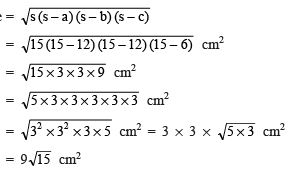Thus, the required area of the triangle = 9√15 cm

,

,

,

,

,

,

,

,

,

,

,

,

,

,

,

,

,

,

,

,

,

;